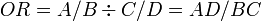# Odds Ratio

This is defined as the ratio of odds of disease among exposed (odds1) and odds of disease among unexposed (odds0).

OR = (odds1) / (odds0)
Disease
Yes No Total
Unexposed A B A+B
Exposed C D C+D
Total A+C B+D N = A+B+C+D

Using the above table, we can calculate the odds ratio using this formula:$OR = A/B \div C/D = AD/BC$

Risk difference percentage (RD%) represents the proportion os cases in the exposed grip that were actually caused by the exposure. This is equal to attributable fraction for exposed (see below). It is calculate as follows:

RD% = (r1 − r0) / r1